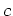Previous: 2.3.5 Hierarchy of Equations Up: II. Nanoscale CMOS Simulation Next: 3.1 Cumulant Closure

# 3. Highest Order Moment Closure

To close the system of equations the highest order moment has to be expressed as a function of the lower order moments. For the numerical properties of the six moments model - especially for robustness - this is an extremely critical issue. In this chapter we review different methods from the literature and propose a new solution to the closure problem.

First, the use of cumulants instead of moments for the description of the distribution function [WSYM98] leads to a generalized Gaussian closure. Second, the maximum entropy principle in the diffusion approximation was applied to solve the closure problem. A third alternative is the use of polynomial approximations which are popular in higher order statistics. Finally, the closure relations proposed in [GKGS01] are considered, where the sixth moment is modeled as a function of the variance and the kurtosis of the distribution function using a real numberas parameter. In the latter case the closure problem is reduced to the choice of. This indeterminacy can be eliminated by requiring consistency with bulk Monte Carlo data.

SubsectionsPrevious: 2.3.5 Hierarchy of Equations Up: II. Nanoscale CMOS Simulation Next: 3.1 Cumulant Closure